# JavaScript Syntax

Syntax of a JavaScript program/code is nothing, it's just a set of rules that defines how JavaScript programs are constructed.

A JavaScript program consists of JavaScript statements that is placed inside the HTML <script> ... </script> tag in a web page.

You are free to place the <script> containing your JavaScript program anywhere within your web page, but to keep it within the <head> tag is the preferred way. Here is the general form shows how script tag plays a role in placing JavaScript code inside it:

```<script ...>
JavaScript code
</script>```

The script tag takes two important attributes:

• language - The language attribute is used to specify what scripting language you are using
• type - The type attribute is what is now recommended to indicate the scripting language in use and its value should be set to "text/javascript"

Now the general form or syntax of your JavaScript code segment will be:

```<script language="javascript" type="text/javascript">
JavaScript code
</script>```

## JavaScript Syntax Example

Here is an example demonstrates syntax of a simple JavaScript example:

```<html>
<body>
<script language="javascript" type="text/javascript">
document.write("Hello JavaScript!")
</script>
</body>
</html>```

Here is the output produced by the above JavaScript program:Here is another JavaScript program, also demonstrates the JavaScript syntax:

```<!DOCTYPE html>
<html>
<head>
<title>JavaScript Syntax</title>
</head>
<body>

<p id="syntax_para1"></p>
<script>
var num1 = 10;
var num2 = 20;
var res = num1 + num2;
document.getElementById("syntax_para1").innerHTML = res;
</script>

</body>
</html>```

In the above example, the variable num1, num2, and num3 are assigned the values 10, 20, and 30. Here is the output of the above JavaScript syntax example: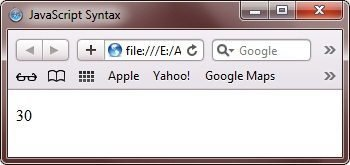To learn more about statement, refer to JavaScript Statements tutorial. Let's take another JavaScript program showing basic syntax of JavaScript program:

```<!DOCTYPE html>
<html>
<head>
<title>JavaScript Basic Syntax</title>
</head>
<body>

<p id="syntax_para2"></p>
<script>
document.getElementById("syntax_para2").innerHTML = 15.23;
</script>

</body>
</html>```

Below is the output of the above JavaScript basic syntax program: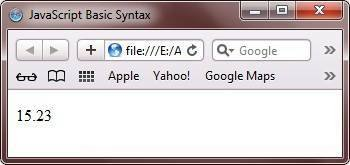Number can be written with or without decimals. To learn more about numbers in JavaScript, refer JavaScript Numbers tutorial. Here is another JavaScript program, for showing general JavaScript syntax:

```<!DOCTYPE html>
<html>
<head>
<title>JavaScript General Syntax</title>
</head>
<body>

<p id="syntax_para3"></p>
<script>
document.getElementById("syntax_para3").innerHTML = 'Codes Cracker';
</script>

</body>
</html>```

Following is the output produced by the above JavaScript general syntax example program:Strings can be written with double or single quotes. To learn more about JavaScript string, refer JavaScript Strings tutorial. Here is one more JavaScript program also illustrates general syntax of a JavaScript program:

```<!DOCTYPE html>
<html>
<head>
<title>Syntax of JavaScript Program</title>
</head>
<body>

<p id="syntax_para4"></p>
<script>
document.getElementById("syntax_para4").innerHTML = 5 * 80;
</script>

</body>
</html>```

This is the output of the above JavaScript syntax program: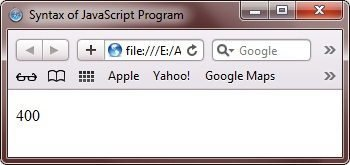Here is another JavaScript program, demonstrating syntax of a JavaScript program:

```<!DOCTYPE html>
<html>
<head>
<title>Syntax of JavaScript Program</title>
</head>
<body>

<p id="syntax_para5"></p>
<script>
var x;
x = 6;
document.getElementById("syntax_para5").innerHTML = x;
</script>

</body>
</html>```

In the above example, x is defined as a variable and then, x is assigned the value of 6. Here is the output: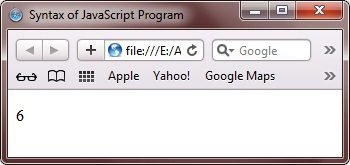To learn in detail about JavaScript variable, then refer to JavaScript Variables tutorial. For now, let's take one more JavaScript program, also demonstrates syntax of a JavaScript. This program uses operators:

```<!DOCTYPE html>
<html>
<head>
<title>JavaScript Syntax Example</title>
</head>
<body>

<p id="syntax_para6"></p>
<script>
var x = 50;
var y = 60;
document.getElementById("syntax_para6").innerHTML = x + y;
</script>

</body>
</html>```

In JavaScript the = operator is used to assign values to variables. Here is the output of the above JavaScript program: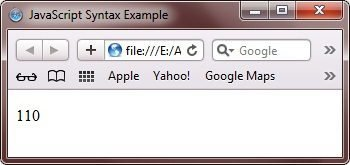Following is another JavaScript program, uses operators to illustrates JavaScript program's syntax:

```<!DOCTYPE html>
<html>
<head>
<title>JavaScript Syntax Program</title>
</head>
<body>

<p id="syntax_para7"></p>
<script>
document.getElementById("syntax_para7").innerHTML = (5 + 6) * 10;
</script>

</body>
</html>```

JavaScript uses arithmetic operators to compute values (just like algebra). It will display the following output in your browser: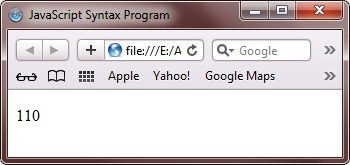Here is another JavaScript program:

```<!DOCTYPE html>
<html>
<head>
<title>JavaScript Program Structure</title>
</head>
<body>

<p id="syntax_para8"></p>
<script>
var num1 = 5 + 6;
var num2 = num1 * 10;
document.getElementById("syntax_para8").innerHTML = num2;
</script>

</body>
</html>```

It will display this output in the browser: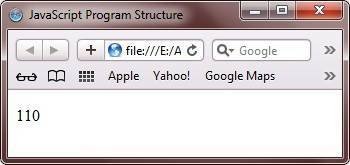Following is an another JavaScript program:

```<!DOCTYPE html>
<html>
<head>
<title>Basic Syntax of JavaScript Program</title>
</head>
<body>

<p id="syntax_para9"></p>
<script>
var x = 5;
// var x = 6; I will not be executed
document.getElementById("syntax_para9").innerHTML = x;
</script>

</body>
</html>```

Following is the output produced by this JavaScript program: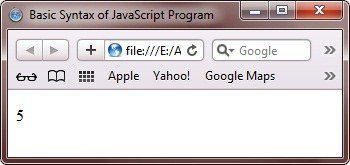Here is another and last JavaScript program, for the complete understanding on syntax of a JavaScript program:

```<!DOCTYPE html>
<html>
<head>
<title>JavaScript Syntax Tutorial</title>
</head>
<body>

<p id="syntax_para101"></p>
<p id="syntax_para102"></p>
<script>
var firstName = "Codes";
var lastname = "Cracker";
document.getElementById("syntax_para101").innerHTML = firstName;
document.getElementById("syntax_para102").innerHTML = lastname;
</script>

</body>
</html>```

Here is the output of the above JavaScript program: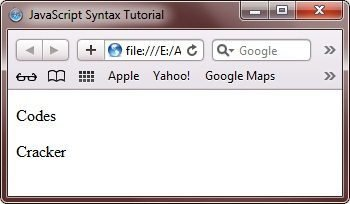Tools
Calculator

Quick Links
Signup - Login - Give Online Test